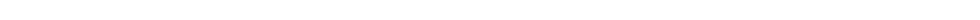### Before I go..

A quick guide to some of the fundamental math behind 3D programming. I won't explain to much as there is plenty of information on the net.

BTW a great article I found explaining the co-ordinate system.

http://creators.xna.com/Headlines/whitepapers/archive/2007/04/26/Shader-Series-supplemental-article-_1320_-Coordinate-systems.aspx

Tips:

1. Vectors:

What is a vector? simple its a point from a origin. Imagine a world with your axis, lets take the 0,0 (or in 3D 0,0,0) as the origin. When we create a new vector we are creating a point a certain distance away from that origin, so (5,2) is a vector 5,2 from the origin.

It is important to grasp this as we may want to manipulate a vector. Imagine we have two vectors in the world, (3,5) and (8,-1). They are both specifying a distance away from the origin, however what if we wanted (3,5) to be a distance away from the other point (8,-1)? Easy, we subtract (8,-1) from (3,5) we are now left with a vector that does just what we want.

2. Matrices

Transforming a point - When we transform a point we are applying one or more transformation matrice to a set of verticies. The order that we apply them in will affect the result.

Rotating a point (vector) - When we rotate a point we are rotating it around the origin, so if a point(vector) is for example (4,2) from the origin then when we rotate it it will be rotated as if orbiting this origin, thus if we want to rotate an object (like a spinning top) and not have it orbiting we need to move this object via translation to the origin, so -(4,2), then apply our rotation, then move the point back to its original position (4,2)

#### 0 comments: